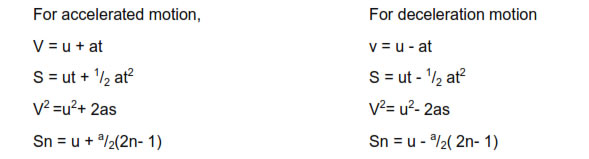# motion in a straight line class 11 notes physics formulas pdf

Motion In A Straight Line Class 11 Notes Physics Formulas Download In Pdf

Chapter 3 Motion in a straight

## Motion in a Straight Line Class 11 Notes Physics Formulas pdf

### Motion:-

Rest and Motion are relative terms, nobody can exist in a state of absolute rest or of absolute motion.

#### One dimensional motion:-

The motion of an object is said to be one dimensional motion if only one out of three coordinates specifying the position of the object change with time. In such a motion an object move along a straight line path.

#### Two dimensional motion:-

The motion of an object is said to be two dimensional motion if two out of three coordinates specifying the position of the object change with time. In such motion the object moves in a plane.

#### Three dimensional motion:-

The motion is said to be three dimensional motion if all the three coordinates specifying the position of an object change with respect to time ,in such a motion an object moves in space.

#### Path length :-

The path length traversed by an object between two points is not the same as the magnitude of displacement always. The displacement depends only on the end points; whereas the path length depends on the actual path. The two quantities are equal only if the object does not change its direction during the course of its motion. In all other cases, the path length is greater than the magnitude of displacement.

Average speed :-

The average speed of an object is greater than or equal to the magnitude of the averagevelocity over a given interval of time. The two are equal only if the path length is equal to the magnitude of the displacement.

#### Displacement

A displacement is the shortest distance from the initial to the final position of a point P. Thus, it is the length of an imaginary straight path, typically distinct from the path actually travelled by P. A displacement vector represents the length and direction of this imaginary straight path.

The magnitude of displacement is less than or equal to the actual distance travelled by the object in the given time interval.

Displacement ≤ Actual distance

#### Speed

It is rate of change of distance covered by the body with respect to time.

Speed = Distance travelled /time taken

Speed is a scalar quantity .
Its unit is meter /sec.
Dimensional formula of speed is [M0L1 T -1 ] .
It is positive or zero but never negative.

#### Uniform Speed:-

If an object covers equal distances in equal intervals of time than the speed of the moving object is called uniform speed. In this type of motion, position – time graph is always a straight line.

#### Instantaneous speed:-

The speed of an object at any particular instant of time is called instantaneous speed. In this measurement, the time ∆t→0. When a body is moving with uniform speed its instantaneous speed = Average speed = uniform speed.

#### Velocity:-

The rate of change of position of an object in a particular direction with respect to time is called velocity. It is equal to the displacement covered by an object per unit time.

Velocity =Displacement /Time

Velocity is a vector quantity
its SI unit is meter per sec.
Its dimensional formula is [M0L1 T -1 ].
It may be negative, positive or zero.
When a body moves in a straight line then the average speed and average velocity are equal.

### Acceleration:-

The rate of change of velocity of an object with respect to time is called its acceleration.

Acceleration = Change in velocity /time taken

It is a vector quantity,
Its SI unit is meter/ sec2
Its dimension is [M0L1 T -2 ].
It may be positive ,negative or zero.

#### Positive Acceleration:-

If the velocity of an object increases with time, its acceleration is positive .

#### Negative Acceleration :-

If the velocity of an object decreases with time, its acceleration is negative . The negative acceleration is also called retardation or deacceleration.

Formulas of uniformly accelerated motion along straight line:

#### Free fall :-

In the absence of the air resistance all bodies fall with the same acceleration towards earth from a small height. This is called free fall. The acceleration with which a body falls is called gravitational acceleration (g).Its value is 9.8 m/sec2 .

#### Relative Motion:-

The rate of change of distance of one object with respect to the other is called relative velocity. The relative velocity of an object B with respect to the object A when both are in motion is the rate of change of position of object B with respect to the object A.

Relative velocity of object A with respect to object B, VAB = VA - VB

When both objects are move in same direction, then the relative velocity of object B with respect to the object A,

VBA = VB - VA

When the object B moves in opposite direction of object A .

VBA = VB + VA### NCERT Books Free Pdf Download for Class 5, 6, 7, 8, 9, 10 , 11, 12 Hindi and English Medium

 Mathematics Biology Psychology Chemistry English Economics Sociology Hindi Business Studies Geography Science Political Science Statistics Physics Accountancy